SSC  >  Thermodynamics - 2

# Thermodynamics - 2

Test Description

## 20 Questions MCQ Test Mock Test Series for SSC JE Mechanical Engineering | Thermodynamics - 2

Thermodynamics - 2 for SSC 2023 is part of Mock Test Series for SSC JE Mechanical Engineering preparation. The Thermodynamics - 2 questions and answers have been prepared according to the SSC exam syllabus.The Thermodynamics - 2 MCQs are made for SSC 2023 Exam. Find important definitions, questions, notes, meanings, examples, exercises, MCQs and online tests for Thermodynamics - 2 below.
Solutions of Thermodynamics - 2 questions in English are available as part of our Mock Test Series for SSC JE Mechanical Engineering for SSC & Thermodynamics - 2 solutions in Hindi for Mock Test Series for SSC JE Mechanical Engineering course. Download more important topics, notes, lectures and mock test series for SSC Exam by signing up for free. Attempt Thermodynamics - 2 | 20 questions in 12 minutes | Mock test for SSC preparation | Free important questions MCQ to study Mock Test Series for SSC JE Mechanical Engineering for SSC Exam | Download free PDF with solutions
 1 Crore+ students have signed up on EduRev. Have you?
Thermodynamics - 2 - Question 1

### Carnot cycle consists of

Detailed Solution for Thermodynamics - 2 - Question 1

Carnot cycle is one of the best-known reversible cycles. The Carnot cycle is composed of four reversible processes.

• Reversible Isothermal Expansion (process 1-2)
• Reversible adiabatic expansion (process 2-3)
• Reversible isothermal compression (process 3-4)
• Reversible adiabatic compression (process 4-1)
•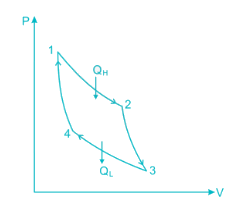•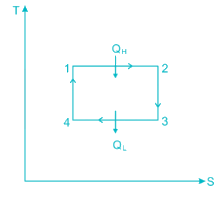Fig. P-V and T-S diagrams of Carnot Cycle
Thermodynamics - 2 - Question 2

### A scientist says that the efficiency of his heat engine which operates at source temperature 127°C and sink temperature 27°C is 26%, then

Detailed Solution for Thermodynamics - 2 - Question 2

Carnot Engine, a reversible heat engine has maximum efficiency when operating between two temperature limits.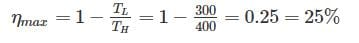So more the 25% efficiency is not possible.

Thermodynamics - 2 - Question 3

### Which one of the following is extensive property of a thermodynamics system

Detailed Solution for Thermodynamics - 2 - Question 3

Intensive Property: These are the properties of system which are independent of mass under consideration. For e.g. Pressure, Temperature, density

Extensive Properties: The properties which depend on the mass of system under consideration.

For e.g Internal Energy, Enthalpy, Volume, Entropy

Note: All specific properties are intensive properties. For e.g. specific volume, specific entropy etc.Since volume depends on mass hence it is extensive property.

Thermodynamics - 2 - Question 4

A heat engine is supplied with 280 kJ/s of heat at a constant fixed temperature of 520 K and heat rejection takes place at 260 K temperature. If the engine is reversible, the heat rejected would be approximate equal to:

Detailed Solution for Thermodynamics - 2 - Question 4

For reversible engine: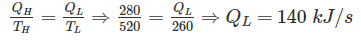Thermodynamics - 2 - Question 5

Properties of substances like pressure, temperature and density, in thermodynamic co-ordinates are _____.

Detailed Solution for Thermodynamics - 2 - Question 5

The thermodynamic properties which depends on the end states only (independent of the path followed) are known as point function like temperature, pressure, density, volume, enthalpy, entropy etc.

The thermodynamic properties which depends on the end states as well as the path followed are known as path function like heat and work.

Thermodynamics - 2 - Question 6

Which of the following represents the perpetual motion of the first kind

Detailed Solution for Thermodynamics - 2 - Question 6

The first law of thermodynamics states that the energy can neither be created nor be destroyed. It can only get transformed from one form to another form. An imaginary device which would produce work continuously without absorbing any energy from its surroundings is called a Perpetual Motion Machine of the First kind, (PMMFK). A PMMFK is a device which violates the first law of thermodynamics. It is impossible to devise a PMMFK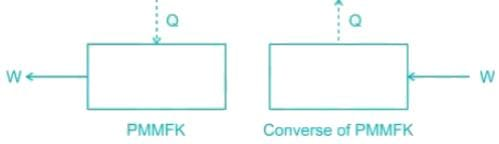The converse of the above statement is also true, i.e., there can be no machine which would continuously consume work without some other form of energy appearing simultaneously.

Thermodynamics - 2 - Question 7

The property of a working substance, which increases or decreases according to the heat supplied or removed in a reversible manner, is called ________.

Detailed Solution for Thermodynamics - 2 - Question 7

Entropy is a property which is a measure of energy dispersion in a system or the irreversibility. Entropy transfer is associated with heat transfer. If the heat is added to the system, then its entropy increases and if heat is lost from the system, its entropy decreases.

Thermodynamics - 2 - Question 8

Dynamic viscosity of most of the gases with rise in temperature...

Detailed Solution for Thermodynamics - 2 - Question 8

The viscosity of liquids decreases with temperature, whereas the viscosity of gases increases with temperatureThis is because in a liquid the molecules possess more energy at higher temperatures, and they can oppose the large cohesive inter-molecular forces more strongly. As a result, the energized liquid molecules can move more freely.

In a gas, on the other hand, the inter-molecular forces are negligible, and the gas molecules at high temperatures move randomly at higher velocities. This results in more molecular collisions per unit volume per unit time and therefore in greater resistance to flow.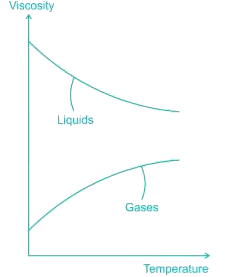Thermodynamics - 2 - Question 9

The standard fixed point of thermometry is

Detailed Solution for Thermodynamics - 2 - Question 9

The triple point of water has a unique value of 273.16 K. At particular value of volume and pressure the triple point of water is always 273.16 K. The melting point of ice and boiling point of water do not have particular values because these points depend on pressure and temperature

Thermodynamics - 2 - Question 10

A closed bottle containing water at 30°C is carried to the moon in a space-ship. If it is placed on the surface of the moon, what will happen to the water as soon as the lid is opened

Detailed Solution for Thermodynamics - 2 - Question 10

Boiling occurs when the vapour pressure of liquid becomes equal to the atmospheric pressure. At the surface of moon, atmospheric pressure is zero, hence boiling point decreases and water begins to boil at 30°C.

So when the bottle is opened, it'll immediately begin to boil, as there's no pressure in the surrounding.

Thermodynamics - 2 - Question 11

The universal gas constant of a gas is the product of molecular weight of the gas and

Detailed Solution for Thermodynamics - 2 - Question 11

R = Universal Gas Constant

Gas Constant = R/M

⇒ R = Gas constant × Molecular weight.

Thermodynamics - 2 - Question 12

One kg of steam sample contains 0.8 kg dry steam; it’s dryness fraction is

Detailed Solution for Thermodynamics - 2 - Question 12

Dryness Fraction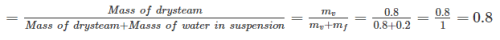Thermodynamics - 2 - Question 13

According to first law of thermodynamics

Detailed Solution for Thermodynamics - 2 - Question 13

According to first Law of thermodynamics, “For a closed system undergoing a cycle, net heat transfer is equal to network transfer.”

ΣQ = ΣW

So, heat and work are mutually convertible.

Thermodynamics - 2 - Question 14

Which relation is the basis of Mollier Diagram?

Detailed Solution for Thermodynamics - 2 - Question 14

From the first and second laws of thermodynamics, the following property relation was obtained,

Tds = dh – vdp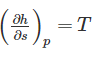This equation forms the basis of the h-s diagram of pure substance, also called the Mollier diagram. The slope of an isobar on the h-s coordinates is equal to the absolute saturation temperature ( tsat + 273) at that pressure.

If the temperature remains constant the slope will remain constant. If the temperature increases, the slope of the isobar will increase.

Thermodynamics - 2 - Question 15

Detailed Solution for Thermodynamics - 2 - Question 15

Steady flow process is a process where: the fluid properties can change from point to point in the control volume but remains the same at any fixed point during the whole process. A steady-flow process is characterized by the following:

• No properties within the control volume change with time. That is mcv = constant; Ecv = constant
• No properties change at the boundaries with time. Thus, the fluid properties at an inlet or exit will remain the same during the whole process.
• The heat and work interactions between a steady-flow system and its surroundings do not change with time.
Thermodynamics - 2 - Question 16

Isothermal compressibility of an ideal gas is

Detailed Solution for Thermodynamics - 2 - Question 16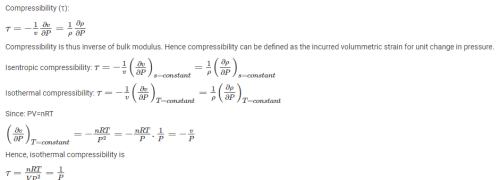Thermodynamics - 2 - Question 17

A process in which no heat is supplied or rejected from the system and entropy is not constant is known as

Detailed Solution for Thermodynamics - 2 - Question 17

A process in which no heat is supplied and rejected is known as adiabatic process. But if entropy is not constant, then this process is called polytropic process

Thermodynamics - 2 - Question 18

Efficiency of a Carnot engine is 75%. If the cycle direction is reverse, COP of the heat pump working on reversed Carnot cycle will be

Detailed Solution for Thermodynamics - 2 - Question 18

Efficiency of Carnot cycle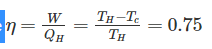By reversing the Carnot cycle we can have either heat pump or refrigerator.

COP of heat pump =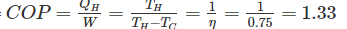Thermodynamics - 2 - Question 19

An isothermal process is governed by

Detailed Solution for Thermodynamics - 2 - Question 19

According to Boyle’s law for a fixed mass of gas at constant temperature, the volume is inversely proportional to the pressure. That means that, for example, if you double the pressure, you will halve the volume. This can express this mathematically as PV = constant.

As isothermal process has constant temperature so governed by Boyle’s law.

Thermodynamics - 2 - Question 20

A Hot Wire Anemometer is used for the measurement of ______.

Detailed Solution for Thermodynamics - 2 - Question 20

An anemometer is a weather monitor instrument used to measure wind speed.

Basic Principle of Hot wire Anemometer

When an electrically heated wire is placed in a flowing gas stream, heat is transferred from the wire to the gas and hence the temperature of the wire reduces, and due to this, the resistance of the wire also changes. This change in resistance of the wire becomes a measure of flow rate.

## Mock Test Series for SSC JE Mechanical Engineering

3 videos|1 docs|55 tests
Information about Thermodynamics - 2 Page
In this test you can find the Exam questions for Thermodynamics - 2 solved & explained in the simplest way possible. Besides giving Questions and answers for Thermodynamics - 2, EduRev gives you an ample number of Online tests for practice

## Mock Test Series for SSC JE Mechanical Engineering

3 videos|1 docs|55 tests(Scan QR code)